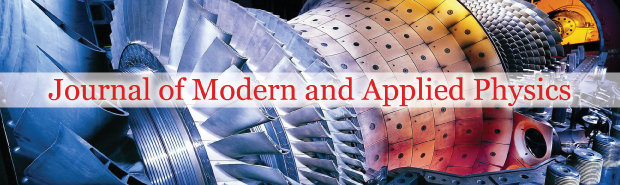All submissions of the EM system will be redirected to Online Manuscript Submission System. Authors are requested to submit articles directly to Online Manuscript Submission System of respective journal.### Premises and simple estimation on proof of the positive mass theorem, and negative matter as unified dark matter and dark energy

Author(s): Yi-Fang Chang*

First, we found that some proofs of the positive mass theorem have all certain premises: an isolated gravitational system and infinite space, but both are all impossible. Therefore, the negative matter cannot be restricted. Second, we discuss some simple estimations of the positive matter theorem, which agree from astronomy to particles. Third, based on Dirac negative energy, Einstein massenergy relation and principle of equivalence, we propose the negative matter as the simplest model of unified dark matter and dark energy. All theories are known, only mass includes positive and negative. Because there is repulsion between positive matter and negative matter, so which is invisible dark matter, and repulsion as dark energy. It may explain many phenomena of dark matter and dark energy. Bondi’s results are wrong. Fourth, we derive that the rotational velocity of galaxy is approximate constant. Assume that dark matter is completely the negative matter, so we may calculate an evolutional ratio between total matter and usual matter from 1 to present 11.82 or 7.88. Fifth, we propose the mechanism of inflation as origin of positive-negative matters created from nothing, whose expansion is exponential due to strong interactions at small microscopic scales. Moreover, we research some theories of the negative matter, and obtain the quantitative relation of the negative mass and the cosmological constant, and predict a judgment test and other possible tests. The negative matter as a candidate of dark matter and dark energy is not only the simplest, and is calculable and testable, and may be changed and developed

PDF

###### Journal of Modern and Applied Physics peer review process verified at publonsTop